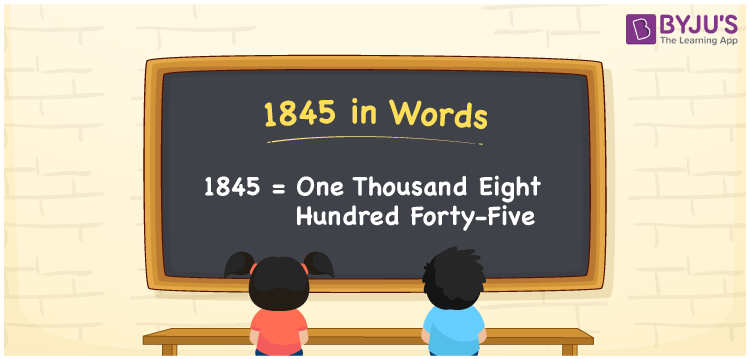# 1845 in Words

1845 in words can be written as One Thousand Eight Hundred Forty-Five. Suppose if you buy a flower vase for Rs. 1845, then you can say that “I bought a flower vase for One Thousand Eight Hundred Forty-Five Rupees”. The place value topic is very important in order to understand the basic Math concepts. Hence, 1845 can be read as “One Thousand Eight Hundred Forty-Five” in words.

 1845 in words One Thousand Eight Hundred Forty-Five One Thousand Eight Hundred Forty-Five in Numbers 1845

## 1845 in English Words## How to Write 1845 in Words?

Learn the applications of numbers in words with the help of the place value chart of 1845 given here.

 Thousands Hundreds Tens Ones 1 8 4 5

The expanded form of 1845 is:

1 x Thousand + 8 × Hundred + 4 × Ten + 5 × One

= 1 x 1000 + 8 × 100 + 4 × 10 + 5 × 1

= 1000 + 800 + 40 + 5

= 1845

= One Thousand Eight Hundred Forty-Five

Therefore, 1845 in words is written as One Thousand Eight Hundred Forty-Five.

1845 is a natural number that precedes 1846 and succeeds 1844.

1845 in words – One Thousand Eight Hundred Forty-Five

Is 1845 an odd number? – Yes

Is 1845 an even number? – No

Is 1845 a perfect square number? – No

Is 1845 a perfect cube number? – No

Is 1845 a prime number? – No

Is 1845 a composite number? – Yes

## Frequently Asked Questions on 1845 in Words

Q1

### Write 1845 in words.

1845 can be written in words as “One Thousand Eight Hundred Forty-Five”.
Q2

### How do you write One Thousand Eight Hundred Forty-Five in numbers?

One Thousand Eight Hundred Forty-Five can be written as 1845 in numbers.
Q3

### Is 1845 a perfect cube number?

No, 1845 is not a perfect cube number as it is not the product of three similar numbers.